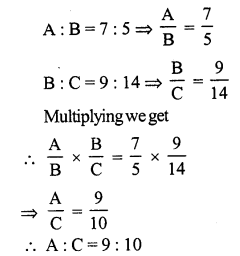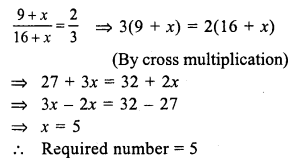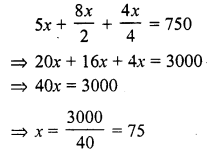# RS Aggarwal Class 7 Solutions Chapter 8 Ratio and Proportion Ex 8A

In this chapter, we provide RS Aggarwal Solutions for Class 7 Chapter 8 Ratio and Proportion Ex 8A for English medium students, Which will very helpful for every student in their exams. Students can download the latest RS Aggarwal Solutions for Class 7 Chapter 8 Ratio and Proportion Ex 8A Maths pdf, free RS Aggarwal Solutions Class 7 Chapter 8 Ratio and Proportion Ex 8A Maths book pdf download. Now you will get step by step solution to each question.

### RS Aggarwal Solutions for Class 7 Chapter 8 Ratio and Proportion Ex 8A Download PDF

Question 1.
Solution:
(i) 24 : 40
HCF of 24, 40 = 8
24 : 40 = 24 ÷ 8 : 40 ÷ 8 = 3 : 5 (Dividing by 8)
(ii) 13.5 : 15 or 135 : 150
HCF of 135 and 150 = 15
135 ÷ 15 : 150 ÷ 15 (Dividing by 15)
= 9 : 10HCF of 25, 65, 80 = 5
Dividing by 5,
5 : 13 : 16

Question 2.
Solution:
(i) 75 paise : 3 rupees = 75 paise : 300 paise
(converting into same kind)
HCF of 75, 300 = 75
75 : 300 = 75 ÷ 75 : 300 ÷ 75 (Dividing by 75) = 1 : 4
(ii) 1 m 5 cm : 63 cm = 105 cm : 63 cm
(converting into same kind)
HCF of 105 and 63 = 21
105 ÷ 21 : 63 ÷ 21 (Dividing by 21)
= 5 : 3
(iii) 1 hour 5 minutes : 45 minutes = 65 minutes : 45 minutes
(converting into minutes)
13 : 9 (dividing by 5)
= 13 : 9
(iv) 8 months : 1 year = 8 months : 12 months
(converting into the same kind)
HCF of 8 and 12 = 4
Dividing by 4
8 ÷ 4 : 12 ÷ 4
= 2 : 3
(v) 2 kg 250 g : 3 kg = 2250 g : 3000 g (converting into the same kind)
HCF of 2250 and 3000 = 750
Dividing by 750,
2250 ÷ 750 : 3000 ÷ 750 = 3 : 4
(vi) 1 km : 750 m = 1000 m : 750 m
(converting into metre)
= 4 : 3 (dividing by 250)
= 4 : 3

Question 3.
Solution:Question 4.
Solution:
A : B = 5 : 8, B : C = 16 : 25Question 5.
Solution:
A : B = 3 : 5, B : C = 10 : 13A : B : C = 6 : 10 : 13

Question 6.
Solution:
A : B = 5 : 6 and B : C = 4 : 7Question 7.
Solution:
Total amount = Rs. 360
Sum of ratios = 7 + 8 = 15Question 8.
Solution:
Total amount = Rs. 880Question 9.
Solution:
Total amount = Rs. 5600
Ratio in A : B : C = 1 : 3 : 4Question 10.
Solution:
Let x be added to each term Then
9 + x : 16 + x = 2 : 3Question 11.
Solution:
Let x be subtracted from each term Then
(17 – x) : (33 – x) = 7 : 15Question 12.
Solution:
Ratio in two numbers = 7 : 11
Let first number = 7x
Then second number = 11x
Then adding 7 to each number, the ratio is 2 : 3
7x+711x+7 = 23
By cross multiplying:
3 (7x + 7) = 2 (11x + 7)
⇒ 21x + 21 = 22x + 14
⇒ 21 – 14 = 22x – 21x
⇒ x = 7
First number = 7x = 7 x 7 = 49
and second number = 11x = 11 x 7 = 77
Hence numbers are 49, 77

Question 13.
Solution:
The ratio in two numbers = 5 : 9
Let the first number = 5x
Then second number = 9x
By subtracting 3 from each number the ratio is 1 : 2
5x–39x–3 = 12
By cross multiplication,
2 (5x – 3) = 1 (9x – 3)
⇒ 10x – 6 = 9x – 3
⇒ 10x – 9x = -3 + 6
⇒ x = 3
First number = 5x = 5 x 3 = 15
and second .number = 9x = 9 x 3 = 27
Hence numbers are 15, 27

Question 14.
Solution:
Ratio in two numbers = 3 : 4
LCM = 180
Let first number = 3x
Then second number = 4x
Now LCM = 3 x 4 x x = 12x
12x = 180
⇒ x = 15
Numbers will be 3 x 15 = 45 and 4 x 15 = 60

Question 15.
Solution:
Ratio in present ages of A and B = 8 : 3
Let A’s age = 8x
Then B’s age = 3x
6 years hence,
A’s age will be = 8x + 6
and B’s will be = 3x + 6
8x+63x+6 = 94
(By cross multiplication)
4 (8x + 6) = 9 (3x + 6)
⇒ 32x + 24 = 27x + 54
⇒ 32x – 27x = 54 – 24
⇒ 5x = 30
⇒ x = 6
A’s present age = 8x = 8 x 6 = 48 years
and B’s age = 3x = 3 x 6 = 18 years

Question 16.
Solution:
Ratio in copper and zinc = 9 : 5
Let alloy = x gmQuestion 17.
Solution:
Ratio in boys and girls = 8 : 3
and total number of girls = 375
Let number of boys = 8x
Then number of girls = 3x
3x = 375
⇒ x = 125
Number of boys = 8x = 8 x 125 = 1000

Question 18.
Solution:
Ratio in income and savings = 11 : 2
Let income = 11x
Then savings = 2x
But savings = Rs. 2500
2x = 2500
⇒ x = 1250
Then income = 1250 x 11 = Rs. 13750
and expenditure = Total income – savings = 13750 – 2500 = Rs. 11250

Question 19.
Solution:
Total amount = Rs. 750
Ratio in rupee, 50 P and 25 P coins =5 : 8 : 4
Let number of rupees = 5x
Number of 50 P coins = 8x
and number of 25 coins = 4x
According to the condition,Number of 1 Re coins = 5x = 5 x 75 = 375
Number of 50 P coins = 8x = 8 x 75 = 600
and number of 25 P coins = 4x = 4 x 75 = 300

Question 20.
Solution:
(4x + 5) : (3x + 11) = 13 : 17
4x+53x+11 = 1317
By cross multiplication,
68x + 85 = 39x + 143
⇒ 68x – 39x = 143 – 85
⇒ 29x = 58
x = 2
Hence x = 2

Question 21.
Solution:
x : y = 3 : 4Question 22.
Solution:
x : y = 6 : 11
xy = 611
Now (8x – 3y) : (3x + 2y)Question 23.
Solution:
Sum of two numbers = 720
Ratio of two numbers = 5 : 7
Let first number = 5x
Then second number = 7x
5x + 7x = 720
⇒ 12x = 720
⇒ x = 60
First number = 5x = 5 x 60 = 300
and second number = 7x = 7 x 60 = 420

Question 24.
Solution:
(i) (5 : 6) or (7 : 9)Question 25.
Solution:
(i) (5 : 6), (8 : 9), (11 : 18)All Chapter RS Aggarwal Solutions For Class 7 Maths

—————————————————————————–

All Subject NCERT Exemplar Problems Solutions For Class 7

All Subject NCERT Solutions For Class 7

*************************************************

I think you got complete solutions for this chapter. If You have any queries regarding this chapter, please comment on the below section our subject teacher will answer you. We tried our best to give complete solutions so you got good marks in your exam.

If these solutions have helped you, you can also share rsaggarwalsolutions.in to your friends.